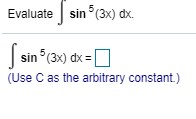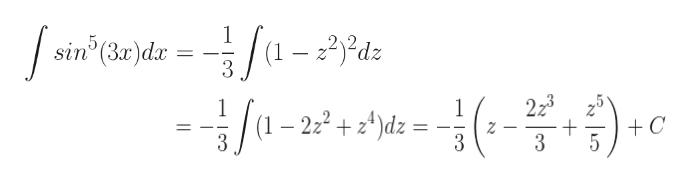Evaluate sin(3x) dx.sin(3x) dx(Use C as the arbitrary constant.)

Questionhelp_outlineImage TranscriptioncloseEvaluate sin(3x) dx. sin(3x) dx (Use C as the arbitrary constant.) fullscreen
Step 1

sin5(3x) = sin4(3x).sin(3x) = [1 – cos2(3x)]2sin(3x)

Step 2

Let cos(3x) = z

Hence, -3sin(3x)dx = dz

Hence, Integrand = sin5(3x)dx  = [1 – cos2(3x)]2sin(3x)dx = - (1 – z2)2dz / 3

Step 3

Please see the wh...help_outlineImage Transcriptionclose1 sin (3r)dar 5 dz 3 223 + 3 --c 1 /1-22+d=- +C Z 3 fullscreen

Want to see the full answer?

See Solution

Want to see this answer and more?

Our solutions are written by experts, many with advanced degrees, and available 24/7

See Solution
Tagged in

Integration